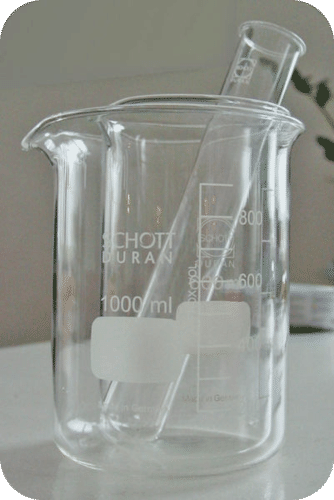# 12.1: Everyday Stoichiometry

$$\newcommand{\vecs}{\overset { \rightharpoonup} {\mathbf{#1}} }$$ $$\newcommand{\vecd}{\overset{-\!-\!\rightharpoonup}{\vphantom{a}\smash {#1}}}$$$$\newcommand{\id}{\mathrm{id}}$$ $$\newcommand{\Span}{\mathrm{span}}$$ $$\newcommand{\kernel}{\mathrm{null}\,}$$ $$\newcommand{\range}{\mathrm{range}\,}$$ $$\newcommand{\RealPart}{\mathrm{Re}}$$ $$\newcommand{\ImaginaryPart}{\mathrm{Im}}$$ $$\newcommand{\Argument}{\mathrm{Arg}}$$ $$\newcommand{\norm}{\| #1 \|}$$ $$\newcommand{\inner}{\langle #1, #2 \rangle}$$ $$\newcommand{\Span}{\mathrm{span}}$$ $$\newcommand{\id}{\mathrm{id}}$$ $$\newcommand{\Span}{\mathrm{span}}$$ $$\newcommand{\kernel}{\mathrm{null}\,}$$ $$\newcommand{\range}{\mathrm{range}\,}$$ $$\newcommand{\RealPart}{\mathrm{Re}}$$ $$\newcommand{\ImaginaryPart}{\mathrm{Im}}$$ $$\newcommand{\Argument}{\mathrm{Arg}}$$ $$\newcommand{\norm}{\| #1 \|}$$ $$\newcommand{\inner}{\langle #1, #2 \rangle}$$ $$\newcommand{\Span}{\mathrm{span}}$$Figure $$\PageIndex{1}$$ (Credit: User:Skatebiker/Wikipedia; Source: http://commons.wikimedia.org/wiki/File:Schott-duran_glassware.PNG; License: Public Domain)

### How much equipment do you need for an experiment?

You are in charge of setting out the lab equipment for a chemistry experiment. If you have twenty students in the lab (and they will be working in teams of two) and the experiment calls for three beakers and two test tubes, how much glassware do you need to set out? Figuring this out involves a type of balanced equation and the sort of calculations that you would do for a chemical reaction.

## Everyday Stoichiometry

You have learned about chemical equations and the techniques used in order to balance them. Chemists use balanced equations to allow them to manipulate chemical reactions in a quantitative manner. Before we look at a chemical reaction, let's consider an equation for the ideal ham sandwich.Figure $$\PageIndex{2}$$: The ideal ham sandwich. (Credit: CK-12 Foundation; Source: CK-12 Foundation; License: CK-12 Curriculum Materials license)

Our ham sandwich is composed of 2 slices of ham $$\left( \ce{H} \right)$$, a slice of cheese $$\left( \ce{C} \right)$$, a slice of tomato $$\left( \ce{T} \right)$$, 5 pickles $$\left( \ce{P} \right)$$, and 2 slices of bread $$\left( \ce{B} \right)$$. The equation for our sandwich is:

$2 \ce{H} + \ce{C} + \ce{T} + 5 \ce{P} + 2 \ce{B} \rightarrow \ce{H_2CTP_5B_2}\nonumber$

Now let us suppose that you are having some friends over and need to make five ham sandwiches. How much of each sandwich ingredient do you need? You take the number of each ingredient required for one sandwich (its coefficient in the above equation) and multiply by five. Using ham and cheese as examples, and using a conversion factor, you can calculate:

$5 \ce{H_2CTP_5B_2} \times \frac{2 \: \ce{H}}{1 \ce{H_2CTP_5B_2}} = 10 \: \ce{H}\nonumber$

$5 \ce{H_2CTP_5B_2} \times \frac{1 \ce{C}}{1 \ce{H_2CTP_5B_2}} = 5 \: \ce{C}\nonumber$

The conversion factors contain the coefficient of each specific ingredient as the numerator and the formula of one sandwich as the denominator. The result is what you would expect. In order to make five ham sandwiches, you need 10 slices of ham and 5 slices of cheese.

This type of calculation demonstrates the use of stoichiometry. Stoichiometry is the calculation of the amount of substances in a chemical reaction from the balanced equation. The sample problem below is another stoichiometry problem involving ingredients of the ideal ham sandwich.

Example $$\PageIndex{1}$$: Ham Sandwich Stoichiometry

Kim looks in the refrigerator and finds that she has 8 slices of ham. In order to make as many sandwiches as possible, how many pickles does she need? Use the equation above.

Solution

Step 1: List the known quantities and plan the problem.

• Have 8 ham slices $$\left( \ce{H} \right)$$
• $$2 \: \ce{H} = 5 \: \ce{P}$$ (conversion factor)

Unknown

• How many pickles (P) needed?

The coefficients for the two reactants (ingredients) are used to make a conversion factor between ham slices and pickles.

Step 2: Solve.

$8 \: \ce{H} \times \frac{5 \: \ce{P}}{2 \: \ce{H}} = 20 \: \ce{P}\nonumber$

Since 5 pickles combine with 2 ham slices in each sandwich, 20 pickles are needed to fully combine with 8 ham slices.

The 8 ham slices will make 4 ham sandwiches. With 5 pickles per sandwich, the 20 pickles are used in the 4 sandwiches.

## Summary

• An example of everyday stoichiometry is given.

## Review

1. A smoothie contains 1 banana (B), 4 strawberries (St), 1 container of yogurt (Y), and 3 ice cubes (Ic). Write a balanced equation to describe the relationship.
2. Write a conversion factor to show the relationship between the number of ice cubes and the number of smoothies produced.
3. How many strawberries would you need to make 12 smoothies?

12.1: Everyday Stoichiometry is shared under a CK-12 license and was authored, remixed, and/or curated by CK-12 Foundation via source content that was edited to conform to the style and standards of the LibreTexts platform; a detailed edit history is available upon request.